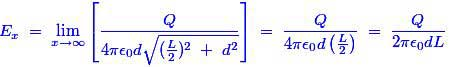# MCQs on Electric Circuits

##### Page 19 of 63. Go to page 1 2 3 4 5 6 7 8 9 10 11 12 13 14 15 16 17 18 19 20 21 22 23 24 25 26 27 28 29 30 31 32 33 34 35 36 37 38 39 40 41 42 43 44 45 46 47 48 49 50 51 52 53 54 55 56 57 58 59 60 61 62 63
01․ The electric field at a point situated at a distance d from straight charged conductor is
proportional to d.
inversely proportional to d.
inversely proportional to d2.
none of above.

The electric field at a point if the conductor length is infinite is as follows;Hence, the electric field is inversely proportional to distance d.

02․ The presence of an uncharged conductor near a charged one increases the
charge of the charged conductor.
capacity of the charged conductor.
potential of the charged conductor.
all of the above.

The presence of an uncharged conductor near a charged conductor would make a condenser or a capacitor. Whenever, the charge increases then the capacity of the charged conductor would also increase.

03․ Which field is associated with the capacitor?
Magnetic.
Electric.
Both of (A) and (B).
None of above.

Capacitor has dielectric medium with it. In dielectric medium, there would be a chance to create an electric field.

04․ An equipotential surface is one which has all points at _____________ potential.
zero.
different.
same.
none of them.

Equipotential surface means potential is constant everywhere. No work is done to move a charge. The equipotential surface are thus orthogonal to the direction of lines of electric field.

05․ A positive and a negative charged conductor are initially 50 mm apart. When they are moved close together so that they are now only 10 mm apart, the force between them will be
5 times smaller than before.
5 times greater than before.
25 times larger than before.
10 times greater than before.

According to the coulombs law the attraction force between a positive and a negative electric charge is inversely proportional to the square of the distance between their center. Here distance is decreased by 5 times, so force would have been decreased by 25 times.

06․ When a dielectric is placed in an electric field the field strength
decreases.
increases.
reduce to zero.
remain unchanged.

An applied electric field would polarize the material by orienting the dipole moments of polar molecules. This dielectric medium decreases the effective electric field intensity between the plates and it would increase the capacitance of the parallel plate capacitor.

07․ Electric flux is proportional to
potential difference between electrodes.
resistivity of medium.
rate of change of potential difference.
rate of change of current.

We know that the formula for capacitance is C = Q / V, this becomes Q = C × V. Hence, this expression is clearly says that the electric flux or charge ‘Q’ is directly proportional to potential difference ‘V’.

08․ An electric field can deflect
gamma-rays.
x-rays.
alpha particles.
neutrons.

X-rays are EM waves and they would not be deflected by electric field. Neutrons are not charged. Alpha particles are charged. Thus, alpha particles could be deflected by electric field, because only charged particles could interact with electric field.

09․ The unit of electric field intensity is
N / C.
N / Ampere.
F / m.
F/ m2.

Electric field intensity is the force experienced by a unit positive charge by another one charge. Hence, E = F / Q . Therefore, the unit of electric field is Newton per Coulomb.

10․ A 2m long conductor, carries a current of 50 A at a magnetic field of 100 × 10 − 3 T. The force on the conductor is
10 N.
100 N.
1000 N.
10000 N.

The magnetic of the force on the conductor, F in the case of conductor of length l meter arranged at right angles to the magnetic field B tesla and carrying a current I, is given by F = BIL = 100×10-3×50×2 = 10 N.

<<<1718192021>>>